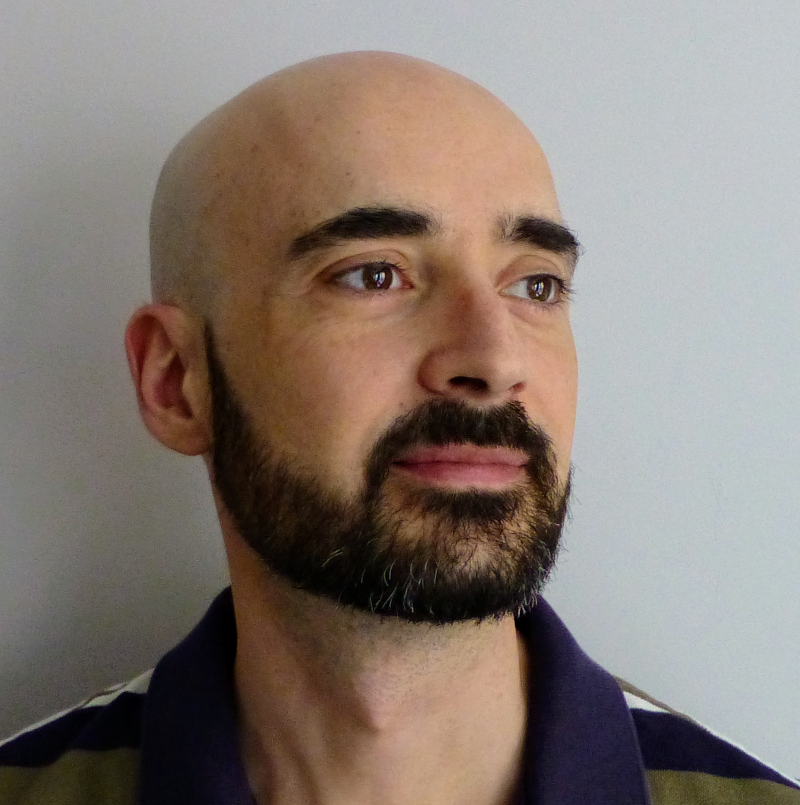Timezone: »

Poster
Optimal Stochastic Non-smooth Non-convex Optimization through Online-to-Non-convex Conversion
Ashok Cutkosky · Harsh Mehta · Francesco Orabona

Wed Jul 26 02:00 PM -- 03:30 PM (PDT) @ Exhibit Hall 1 #623
We present new algorithms for optimizing non-smooth, non-convex stochastic objectives based on a novel analysis technique. This improves the current best-known complexity for finding a $(\delta,\epsilon)$-stationary point from $O(\epsilon^{-4}\delta^{-1})$ stochastic gradient queries to $O(\epsilon^{-3}\delta^{-1})$, which we also show to be optimal. Our primary technique is a reduction from non-smooth non-convex optimization to *online learning*, after which our results follow from standard regret bounds in online learning. For *deterministic and second-order smooth* objectives, applying more advanced optimistic online learning techniques enables a new complexity of $O(\epsilon^{-1.5}\delta^{-0.5})$. Our improved non-smooth analysis also immediately recovers all optimal or best-known results for finding $\epsilon$ stationary points of smooth or second-order smooth objectives in both stochastic and deterministic settings.

#### Author Information

##### Ashok Cutkosky (Boston University)